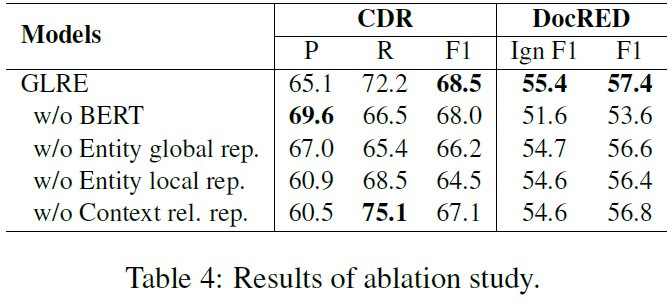## Background

### 论文动机：

1. 文档关系抽取的难点在于，在一篇文档中，其包含多个实体，每个实体（entities）拥有多个处于不同上下文的提及（mentions）。为了识别出跨句子实体之间的关系，抽取模型需要能够建模文档中==多个实体之间的复杂交互以及综合利用实体的多个提及的上下文信息==。

2. 有一个重要的点：there is still a big gap between word representations and relation prediction. 这也是我一直的疑惑，单词的表示和关系的预测 可以说是关系不大的；

3. 噪声问题，以往模型无差别地整合所有信息（the irrelevant information would be involved as noise and damages the prediction accuracy）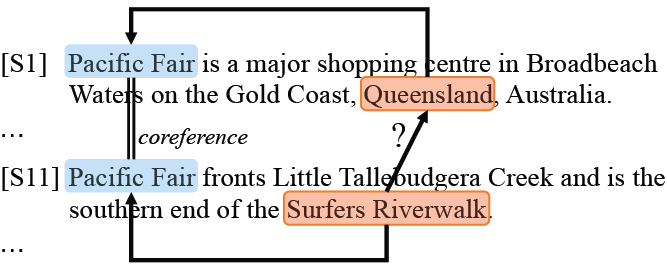### 论文贡献：

1. 如何建模文档复杂的语义信息？

• BERT <=> Capture semantic feature and common-sense knowledge
• 启发式规则构建异构图建模提及、实体、句子之间的语义交互信息。
2. 如何有效的学习实体的多粒度表示？

• Global representation layer

实体的全局语义信息使用L层R-GCN来构建的异构图进行卷积，得到实体的全局表示；

• Local representation layer

局部表示主要是考虑了实体在==不同目标实体对==预测时的==局部偏向性语义信息==；

3. 如何利用文档的主题信息 ？

• 这点很有意思啊，文档的主题信息可以辅助关系的判断；

### Model（loc representation很新颖）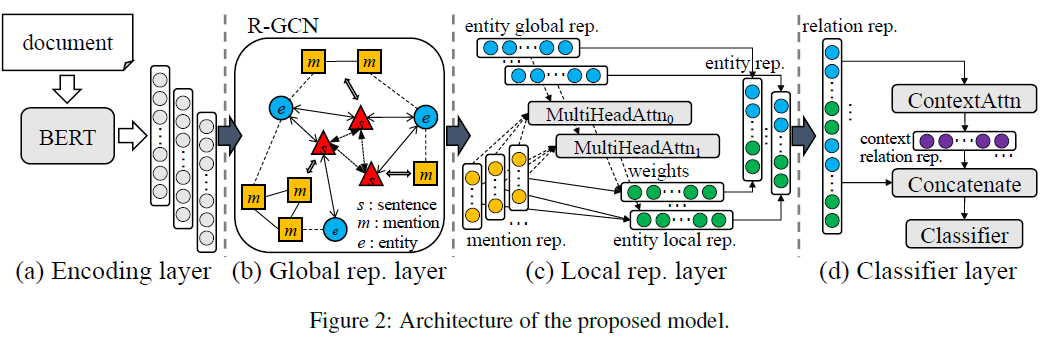• Encoding Layer

$Doc = [w_1,w_2,…,w_k]$ , $w_j$ is the $j^{th}$ word in document.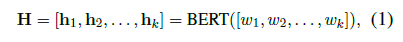• Global Layer

每个类型的节点都包含着全局语义信息

Construct a global heterogeneous graph, with different types of nodes and edges.

这一思想来自 这篇论文 “Connecting the dots: Document-level neural relation extraction with edge-oriented graphs” ：连接不同类型的边和节点，以此捕获不同的依赖关系（共现，共指，顺序[order dependencies]依赖）

三种类型的节点： mention nodes (M), entity nodes (E), sentence nodes(S);

五种类型的边： M-M edges, M-E edges, M-S edges, E-S edges, S-S edges.

Different from GCN, R-GCN considers various types of edges and can better model multi-relational graphs.

An L-layer stacked R-GCN: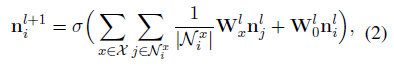得到 entity global representations : $e_{i}^{glo}$

• Local Layer（==关键==创新）

出发点: 在不同的实体对中，每一个实体都有不同的 local representations.(这个也很好理解，因为即使是同一实体在不同上下文中或者不同实体对中的表示按道理应该是有差异的)

怎么解决：多头注意力机制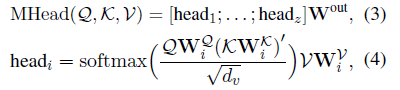• $Q$ is related to the entity global rep.（resentations）
• $K$ is related to the inital sentence node rep.
• $V$ is related to the inital mention node rep.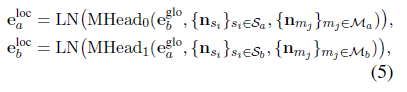• $\mathcal{M}_a$ 表示 实体 $a$ 的相关提及集合；

• $\mathcal{S}_a$ 表示相应的 句子节点 （which each mention node in $\mathcal{M}_a$ is located）

局部表示建模了不同目标实体对预测时的局部偏向性语义信息，利用多头注意力机制针对具体实体对有选择性的聚合多个提及的表示；

直觉上看，如果在同一句话中包含关于实体 a 和实体 b 的mentions ,$m_a$和 $m_b$, 那么提及节点表示（mention node representations $n_{m_{a}}$,$n_{m_b}$）对最终的预测两实体 $a,b$ 间的关系有更大的贡献，那么在生成$e_a^{loc}$,$e_b^{loc}$也会赋予更大的注意力权重;

更一般的说，如果包含 $m_a$ 的sentence node 和 $e_b^{glo}$语义相似度越高（如下红框）：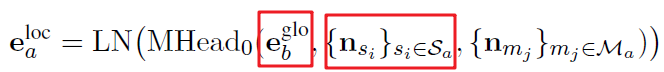表示这一sentence和$m_b$在语义上越关联，那么 $n_{m_{a}}$对生成的$e_a^{loc}$的贡献越大；

• Classifier Layer

e = [global rep., local rep.,relative distance rep.]​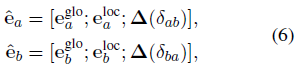$\delta_{ab}$表示 实体a 和实体 b的相对距离，后续被统一到一个区间 bin 中；

concatenate the final representation：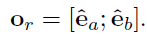document’s 主题信息 会 暗示 可能的关系类型；

Thus we use self-attention to capture context relation representions：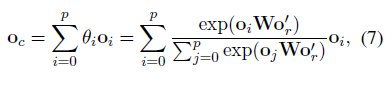$o_i$（$o_j$） is the relation representation of the $i^{th}$ / $j^{th}$ entity pair.

==考虑到一组实体对可能会有不止一类关系，我们将多分类问题转换为多个二分类问题（multiple binary classifiction）==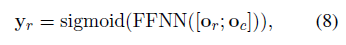Loss function：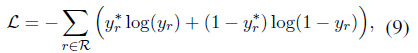## Experiment

• 数据集

• DocRED. / CDR.
• 实验结果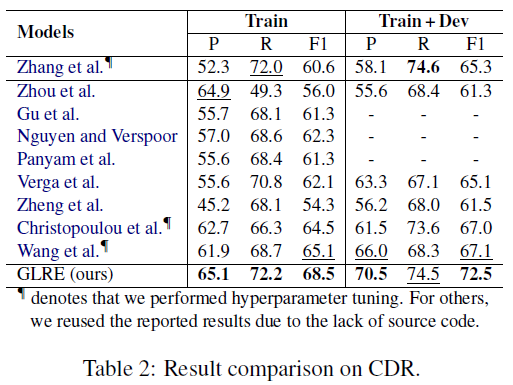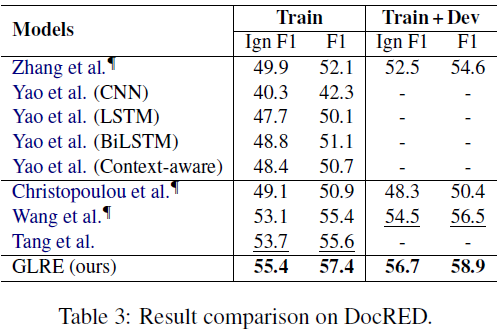• 实验发现

实体距离-Entity distance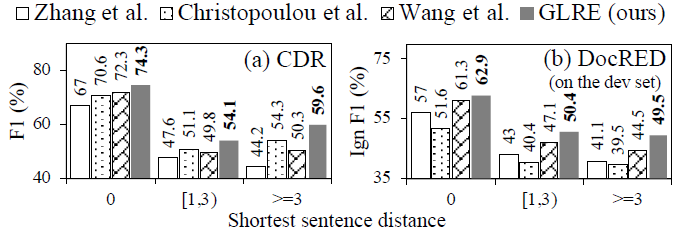• GLRE在实体距离（which is defined as the shortest sentence distance between all mentions of two entities）>=3的情况下表现最好
• global heterogeneous graph : 能够有效建模不同类型节点之间的语义交互信息；
• entity local representation：降低长距离上的不同提及带来的噪声上下文；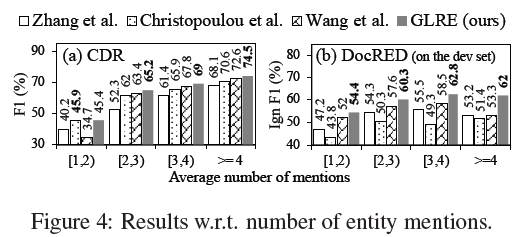• 实体提及数量- number of entity mentions

对于同一实体，提及的数量越多越好，但是随着提及数量的增加，带来的noisy context不可忽视（所以我们能看到随着实体提及的增加，$Ign F1$并不是一直在提升/提升很有限）

• 消融实验